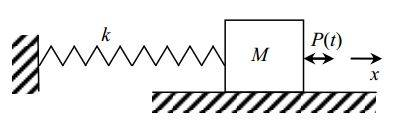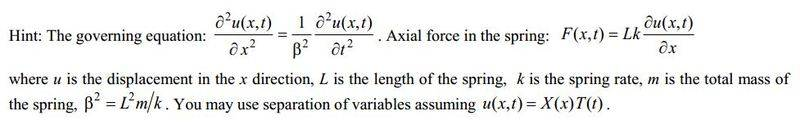# Find the natural frequency of a spring

## Homework StatementConsider the spring-mass system shown. A dynamic load, P(t), is applied with a frequency of 300 rpm. The helical compression spring is made of a music wire. When installed, the spring force is to vary between 100 and 500 N while the spring height varies over a range of 10 mm. The object attached to its end has a mass of 1.7 kg.

Derive an expression for natural frequency.

## Homework Equations

None. There might be some unnecessary information in the question, I'm not sure. This is an example from my machine design course.

## The Attempt at a Solution

I know that a spring's natural frequency is 1/2∏ √(k/m). But I don't know this applies to this question.

Thanks!

## The Attempt at a Solution

BruceW
Homework Helper
I know that a spring's natural frequency is 1/2∏ √(k/m). But I don't know this applies to this question.
That's right. But the question is not so easy, because they are not giving you k directly. But they have told you how the force varies, as the compression of the spring varies. So make use of what they are telling you. What equations do you know that are related to the force and compression of a spring?

That's right. But the question is not so easy, because they are not giving you k directly. But they have told you how the force varies, as the compression of the spring varies. So make use of what they are telling you. What equations do you know that are related to the force and compression of a spring?

Well, first I have no idea what the force function is. Is it sinusoidal? And formulas:

F = kx
k = Gd^4 / 8D^3n

What is the mass? Is it the mass of the spring + mass of the brick?

And if I understood correctly, the external force applied has no effect on the natural frequency. Right?

EDIT: Oh, also this was given as well. But I have no idea at all how to use it.haruspex
Homework Helper
Gold Member
2020 AwardThat puts a very different slant on the problem. You cannot deduce k directly from the extension and tension data given. The spring has mass, so a wave arises within the spring. The applied load frequency interacts with this to affect the tension.
You will need to obtain solutions to the differential equation. You understand separation of variables?

That puts a very different slant on the problem. You cannot deduce k directly from the extension and tension data given. The spring has mass, so a wave arises within the spring. The applied load frequency interacts with this to affect the tension.
You will need to obtain solutions to the differential equation. You understand separation of variables?

Actually not quite. Are we trying to obtain F(x,t)?

And what about my other questions:

What is the mass? Is it the mass of the spring + mass of the brick?

And if I understood correctly, the external force applied has no effect on the natural frequency. Right?

Thanks for any help!

haruspex
Homework Helper
Gold Member
2020 Award
Are we trying to obtain F(x,t)?
Yes.
What is the mass? Is it the mass of the spring + mass of the brick?
Your problem statement only mentions the mass of the block, M, but the 'hint' says the spring also has mass, m.
And if I understood correctly, the external force applied has no effect on the natural frequency.
That's true, but how are you going to calculate it? You are not told k, and you cannot deduce it directly from "the spring force is to vary between 100 and 500 N while the spring height varies over a range of 10 mm". That piece of information only relates to the forced mode of oscillation. So you need to solve the equations for this forced mode, use that information to determine k, and thus find the natural frequency.

Yes.

Your problem statement only mentions the mass of the block, M, but the 'hint' says the spring also has mass, m.

That's true, but how are you going to calculate it? You are not told k, and you cannot deduce it directly from "the spring force is to vary between 100 and 500 N while the spring height varies over a range of 10 mm". That piece of information only relates to the forced mode of oscillation. So you need to solve the equations for this forced mode, use that information to determine k, and thus find the natural frequency.

Thanks. This helps a lot. These might be fundamental questions but I'll ask them anyway:

1) How do I obtain F(x,t)?
2) After I obtain F(x,t), how do I go to k from that? I thought F = kx but in this case F is a function.

haruspex
Homework Helper
Gold Member
2020 Award
In the 'governing' differential equation, make the substitution for u they suggest. Obtain a general solution and apply the boundary conditions. Can you see what the boundary conditions are?

In the 'governing' differential equation, make the substitution for u they suggest. Obtain a general solution and apply the boundary conditions. Can you see what the boundary conditions are?
I make the substitution and apply the multiplication derivative rule?

I know that one boundary condition is u(0,t)=0. Are there more?

haruspex